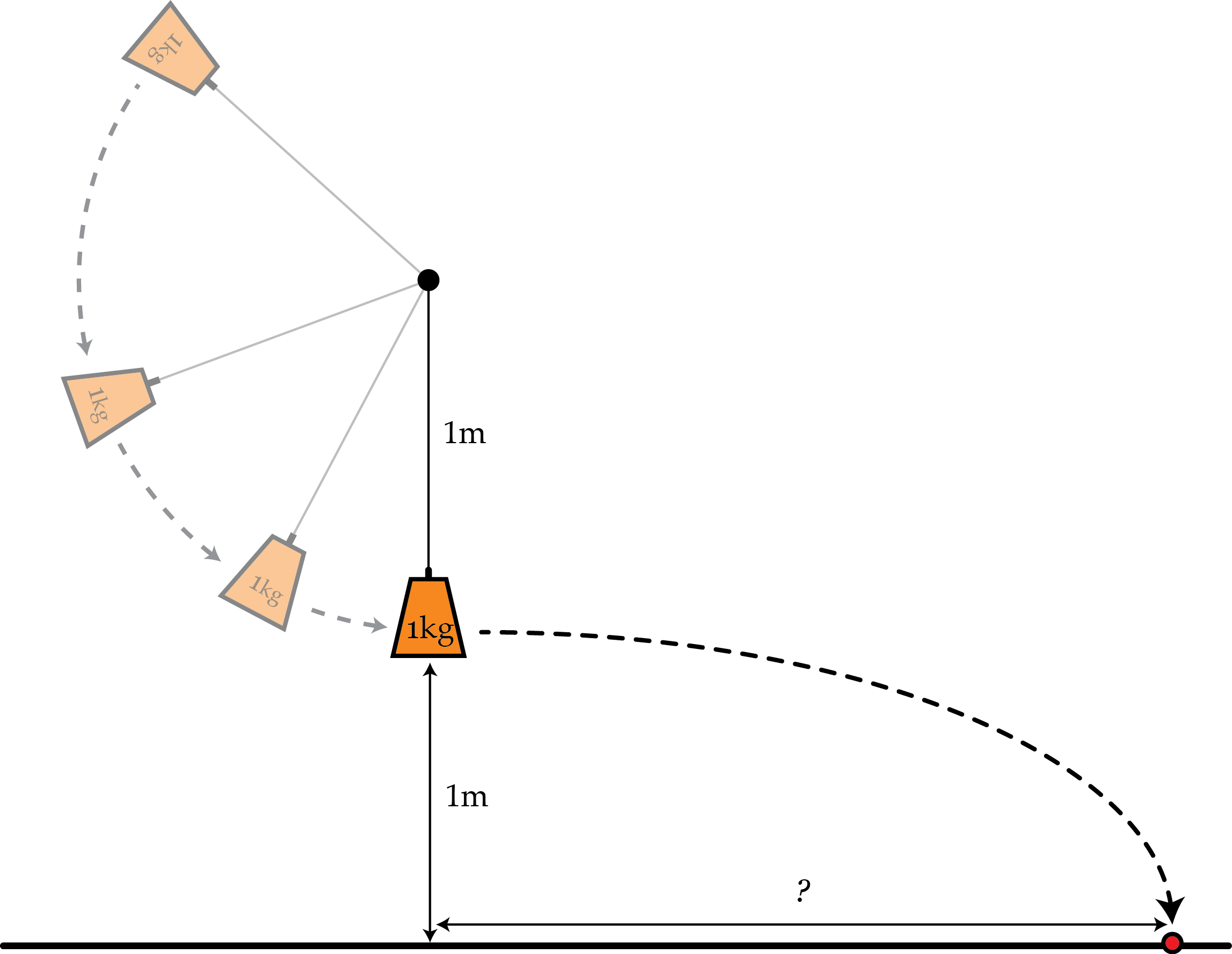# Duck, incoming rock!

You tie a $1~\mbox{kg}$ mass to the end of a $1~\mbox{m}$ long string. The string has a breaking tension of $200~\mbox{N}$ and you swing it in a vertical circle faster and faster. At the very bottom of one swing the string breaks and the mass flies off horizontally from a height of $1~\mbox{m}$. How far has the mass gone horizontally in meters when it hits the ground?Details and assumptions

• The acceleration of gravity is $-9.8~\mbox{m/s}^2$.
×